/*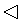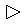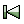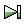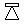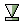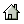*/

## DEFINITIONS

This source file includes following definitions.
```/* included by thread.c */

static VALUE rb_cMutex, rb_cQueue, rb_cSizedQueue, rb_cConditionVariable;
static VALUE rb_eClosedQueueError;

/* Mutex */

typedef struct rb_mutex_struct {
struct rb_mutex_struct *next_mutex;
int cond_waiting;
int allow_trap;
} rb_mutex_t;

#if defined(HAVE_WORKING_FORK)
static void rb_mutex_abandon_all(rb_mutex_t *mutexes);
#endif
static const char* rb_mutex_unlock_th(rb_mutex_t *mutex, rb_thread_t volatile *th);

/*
*  Document-class: Mutex
*
*  Mutex implements a simple semaphore that can be used to coordinate access to
*  shared data from multiple concurrent threads.
*
*  Example:
*
*    semaphore = Mutex.new
*
*      semaphore.synchronize {
*        # access shared resource
*      }
*    }
*
*      semaphore.synchronize {
*        # access shared resource
*      }
*    }
*
*/

#define GetMutexPtr(obj, tobj) \
TypedData_Get_Struct((obj), rb_mutex_t, &mutex_data_type, (tobj))

#define mutex_mark NULL

static void
mutex_free(void *ptr)
{
if (ptr) {
rb_mutex_t *mutex = ptr;
if (mutex->th) {
/* rb_warn("free locked mutex"); */
const char *err = rb_mutex_unlock_th(mutex, mutex->th);
if (err) rb_bug("%s", err);
}
native_mutex_destroy(&mutex->lock);
native_cond_destroy(&mutex->cond);
}
ruby_xfree(ptr);
}

static size_t
mutex_memsize(const void *ptr)
{
return sizeof(rb_mutex_t);
}

static const rb_data_type_t mutex_data_type = {
"mutex",
{mutex_mark, mutex_free, mutex_memsize,},
0, 0, RUBY_TYPED_FREE_IMMEDIATELY
};

VALUE
rb_obj_is_mutex(VALUE obj)
{
if (rb_typeddata_is_kind_of(obj, &mutex_data_type)) {
return Qtrue;
}
else {
return Qfalse;
}
}

static VALUE
mutex_alloc(VALUE klass)
{
VALUE obj;
rb_mutex_t *mutex;

obj = TypedData_Make_Struct(klass, rb_mutex_t, &mutex_data_type, mutex);
native_mutex_initialize(&mutex->lock);
native_cond_initialize(&mutex->cond, RB_CONDATTR_CLOCK_MONOTONIC);
return obj;
}

/*
*  call-seq:
*     Mutex.new   -> mutex
*
*  Creates a new Mutex
*/
static VALUE
mutex_initialize(VALUE self)
{
return self;
}

VALUE
rb_mutex_new(void)
{
return mutex_alloc(rb_cMutex);
}

/*
* call-seq:
*    mutex.locked?  -> true or false
*
* Returns +true+ if this lock is currently held by some thread.
*/
VALUE
rb_mutex_locked_p(VALUE self)
{
rb_mutex_t *mutex;
GetMutexPtr(self, mutex);
return mutex->th ? Qtrue : Qfalse;
}

static void
{
rb_mutex_t *mutex;
GetMutexPtr(self, mutex);

if (th->keeping_mutexes) {
mutex->next_mutex = th->keeping_mutexes;
}
th->keeping_mutexes = mutex;
}

/*
* call-seq:
*    mutex.try_lock  -> true or false
*
* Attempts to obtain the lock and returns immediately. Returns +true+ if the
* lock was granted.
*/
VALUE
rb_mutex_trylock(VALUE self)
{
rb_mutex_t *mutex;
VALUE locked = Qfalse;
GetMutexPtr(self, mutex);

native_mutex_lock(&mutex->lock);
if (mutex->th == 0) {
mutex->th = th;
locked = Qtrue;

mutex_locked(th, self);
}
native_mutex_unlock(&mutex->lock);

return locked;
}

static int
lock_func(rb_thread_t *th, rb_mutex_t *mutex, int timeout_ms)
{
int interrupted = 0;
int err = 0;

mutex->cond_waiting++;
for (;;) {
if (!mutex->th) {
mutex->th = th;
break;
}
if (RUBY_VM_INTERRUPTED(th)) {
interrupted = 1;
break;
}
if (err == ETIMEDOUT) {
interrupted = 2;
break;
}

if (timeout_ms) {
struct timespec timeout_rel;
struct timespec timeout;

timeout_rel.tv_sec = 0;
timeout_rel.tv_nsec = timeout_ms * 1000 * 1000;
timeout = native_cond_timeout(&mutex->cond, timeout_rel);
err = native_cond_timedwait(&mutex->cond, &mutex->lock, &timeout);
}
else {
native_cond_wait(&mutex->cond, &mutex->lock);
err = 0;
}
}
mutex->cond_waiting--;

return interrupted;
}

static void
lock_interrupt(void *ptr)
{
rb_mutex_t *mutex = (rb_mutex_t *)ptr;
native_mutex_lock(&mutex->lock);
if (mutex->cond_waiting > 0)
native_mutex_unlock(&mutex->lock);
}

/*
* At maximum, only one thread can use cond_timedwait and watch deadlock
* introduces new race conditions. [Bug #6278] [ruby-core:44275]
*/

/*
* call-seq:
*    mutex.lock  -> self
*
* Attempts to grab the lock and waits if it isn't available.
*/
VALUE
rb_mutex_lock(VALUE self)
{
rb_mutex_t *mutex;
GetMutexPtr(self, mutex);

/* When running trap handler */
rb_raise(rb_eThreadError, "can't be called from trap context");
}

if (rb_mutex_trylock(self) == Qfalse) {
if (mutex->th == th) {
}

while (mutex->th != th) {
int interrupted;
volatile int timeout_ms = 0;
struct rb_unblock_callback oldubf;

set_unblock_function(th, lock_interrupt, mutex, &oldubf, FALSE);
th->locking_mutex = self;

native_mutex_lock(&mutex->lock);
th->vm->sleeper++;
/*
* Carefully! while some contended threads are in lock_func(),
* vm->sleepr is unstable value. we have to avoid both deadlock
* and busy loop.
*/
timeout_ms = 100;
}

GVL_UNLOCK_BEGIN();
interrupted = lock_func(th, mutex, (int)timeout_ms);
native_mutex_unlock(&mutex->lock);
GVL_UNLOCK_END();

reset_unblock_function(th, &oldubf);

th->locking_mutex = Qfalse;
if (mutex->th && interrupted == 2) {
}
th->status = prev_status;
}
th->vm->sleeper--;

if (mutex->th == th) mutex_locked(th, self);

if (interrupted) {
RUBY_VM_CHECK_INTS_BLOCKING(th);
}
}
}
return self;
}

/*
* call-seq:
*    mutex.owned?  -> true or false
*
* Returns +true+ if this lock is currently held by current thread.
*/
VALUE
rb_mutex_owned_p(VALUE self)
{
VALUE owned = Qfalse;
rb_mutex_t *mutex;

GetMutexPtr(self, mutex);

if (mutex->th == th)
owned = Qtrue;

return owned;
}

static const char *
{
const char *err = NULL;

native_mutex_lock(&mutex->lock);

if (mutex->th == 0) {
err = "Attempt to unlock a mutex which is not locked";
}
else if (mutex->th != th) {
err = "Attempt to unlock a mutex which is locked by another thread";
}
else {
mutex->th = 0;
if (mutex->cond_waiting > 0)
native_cond_signal(&mutex->cond);
}

native_mutex_unlock(&mutex->lock);

if (!err) {
rb_mutex_t *volatile *th_mutex = &th->keeping_mutexes;
while (*th_mutex != mutex) {
th_mutex = &(*th_mutex)->next_mutex;
}
*th_mutex = mutex->next_mutex;
mutex->next_mutex = NULL;
}

return err;
}

/*
* call-seq:
*    mutex.unlock    -> self
*
* Releases the lock.
*/
VALUE
rb_mutex_unlock(VALUE self)
{
const char *err;
rb_mutex_t *mutex;
GetMutexPtr(self, mutex);

return self;
}

#if defined(HAVE_WORKING_FORK)
static void
{
if (th->keeping_mutexes) {
rb_mutex_abandon_all(th->keeping_mutexes);
}
th->keeping_mutexes = NULL;
}

static void
{
rb_mutex_t *mutex;

if (!th->locking_mutex) return;

GetMutexPtr(th->locking_mutex, mutex);
if (mutex->th == th)
rb_mutex_abandon_all(mutex);
th->locking_mutex = Qfalse;
}

static void
rb_mutex_abandon_all(rb_mutex_t *mutexes)
{
rb_mutex_t *mutex;

while (mutexes) {
mutex = mutexes;
mutexes = mutex->next_mutex;
mutex->th = 0;
mutex->next_mutex = 0;
}
}
#endif

static VALUE
rb_mutex_sleep_forever(VALUE time)
{
sleep_forever(GET_THREAD(), 1, 0); /* permit spurious check */
return Qnil;
}

static VALUE
rb_mutex_wait_for(VALUE time)
{
struct timeval *t = (struct timeval *)time;
sleep_timeval(GET_THREAD(), *t, 0); /* permit spurious check */
return Qnil;
}

VALUE
rb_mutex_sleep(VALUE self, VALUE timeout)
{
time_t beg, end;
struct timeval t;

if (!NIL_P(timeout)) {
t = rb_time_interval(timeout);
}
rb_mutex_unlock(self);
beg = time(0);
if (NIL_P(timeout)) {
rb_ensure(rb_mutex_sleep_forever, Qnil, rb_mutex_lock, self);
}
else {
rb_ensure(rb_mutex_wait_for, (VALUE)&t, rb_mutex_lock, self);
}
end = time(0) - beg;
return INT2FIX(end);
}

/*
* call-seq:
*    mutex.sleep(timeout = nil)    -> number
*
* Releases the lock and sleeps +timeout+ seconds if it is given and
* non-nil or forever.  Raises +ThreadError+ if +mutex+ wasn't locked by
*
* When the thread is next woken up, it will attempt to reacquire
* the lock.
*
* Note that this method can wakeup without explicit Thread#wakeup call.
* For example, receiving signal and so on.
*/
static VALUE
mutex_sleep(int argc, VALUE *argv, VALUE self)
{
VALUE timeout;

rb_scan_args(argc, argv, "01", &timeout);
return rb_mutex_sleep(self, timeout);
}

/*
* call-seq:
*    mutex.synchronize { ... }    -> result of the block
*
* Obtains a lock, runs the block, and releases the lock when the block
* completes.  See the example under +Mutex+.
*/

VALUE
rb_mutex_synchronize(VALUE mutex, VALUE (*func)(VALUE arg), VALUE arg)
{
rb_mutex_lock(mutex);
return rb_ensure(func, arg, rb_mutex_unlock, mutex);
}

/*
* call-seq:
*    mutex.synchronize { ... }    -> result of the block
*
* Obtains a lock, runs the block, and releases the lock when the block
* completes.  See the example under +Mutex+.
*/
static VALUE
rb_mutex_synchronize_m(VALUE self, VALUE args)
{
if (!rb_block_given_p()) {
rb_raise(rb_eThreadError, "must be called with a block");
}

return rb_mutex_synchronize(self, rb_yield, Qundef);
}

void rb_mutex_allow_trap(VALUE self, int val)
{
rb_mutex_t *m;
GetMutexPtr(self, m);

m->allow_trap = val;
}

/* Queue */

enum {
QUEUE_QUE,
QUEUE_WAITERS,
SZQUEUE_WAITERS,
SZQUEUE_MAX,
END_QUEUE
};

#define QUEUE_CLOSED          FL_USER5

#define GET_QUEUE_QUE(q)        get_array((q), QUEUE_QUE)
#define GET_QUEUE_WAITERS(q)    get_array((q), QUEUE_WAITERS)
#define GET_SZQUEUE_WAITERS(q)  get_array((q), SZQUEUE_WAITERS)
#define GET_SZQUEUE_MAX(q)      RSTRUCT_GET((q), SZQUEUE_MAX)
#define GET_SZQUEUE_ULONGMAX(q) NUM2ULONG(GET_SZQUEUE_MAX(q))

static VALUE
ary_buf_new(void)
{
return rb_ary_tmp_new(1);
}

static VALUE
get_array(VALUE obj, int idx)
{
VALUE ary = RSTRUCT_GET(obj, idx);
if (!RB_TYPE_P(ary, T_ARRAY)) {
rb_raise(rb_eTypeError, "%+"PRIsVALUE" not initialized", obj);
}
return ary;
}

static void
{

}
}

static void
{
long i;

for (i=0; i<RARRAY_LEN(list); i++) {
}
rb_ary_clear(list);
}

static unsigned long
queue_length(VALUE self)
{
VALUE que = GET_QUEUE_QUE(self);
return RARRAY_LEN(que);
}

static unsigned long
queue_num_waiting(VALUE self)
{
VALUE waiters = GET_QUEUE_WAITERS(self);
return RARRAY_LEN(waiters);
}

static unsigned long
szqueue_num_waiting_producer(VALUE self)
{
VALUE waiters = GET_SZQUEUE_WAITERS(self);
return RARRAY_LEN(waiters);
}

static int
queue_closed_p(VALUE self)
{
return FL_TEST_RAW(self, QUEUE_CLOSED) != 0;
}

static void
raise_closed_queue_error(VALUE self)
{
rb_raise(rb_eClosedQueueError, "queue closed");
}

static VALUE
queue_closed_result(VALUE self)
{
assert(queue_length(self) == 0);
return Qnil;
}

static VALUE
queue_do_close(VALUE self, int is_szq)
{
if (!queue_closed_p(self)) {
FL_SET(self, QUEUE_CLOSED);

if (queue_num_waiting(self) > 0) {
VALUE waiters = GET_QUEUE_WAITERS(self);
}

if (is_szq && szqueue_num_waiting_producer(self) > 0) {
VALUE waiters = GET_SZQUEUE_WAITERS(self);
}
}

return self;
}

/*
*  Document-class: Queue
*
*  The Queue class implements multi-producer, multi-consumer queues.
*  It is especially useful in threaded programming when information
*  must be exchanged safely between multiple threads. The Queue class
*  implements all the required locking semantics.
*
*  The class implements FIFO type of queue. In a FIFO queue, the first
*
*  Example:
*
*      queue = Queue.new
*
*        5.times do |i|
*           sleep rand(i) # simulate expense
*           queue << i
*           puts "#{i} produced"
*        end
*      end
*
*        5.times do |i|
*           value = queue.pop
*           sleep rand(i/2) # simulate expense
*           puts "consumed #{value}"
*        end
*      end
*
*/

/*
* Document-method: Queue::new
*
* Creates a new queue instance.
*/

static VALUE
rb_queue_initialize(VALUE self)
{
RSTRUCT_SET(self, QUEUE_QUE, ary_buf_new());
RSTRUCT_SET(self, QUEUE_WAITERS, ary_buf_new());
return self;
}

static VALUE
queue_do_push(VALUE self, VALUE obj)
{
if (queue_closed_p(self)) {
raise_closed_queue_error(self);
}
rb_ary_push(GET_QUEUE_QUE(self), obj);
return self;
}

/*
* Document-method: Queue#close
* call-seq:
*   close
*
* Closes the queue. A closed queue cannot be re-opened.
*
* After the call to close completes, the following are true:
*
* - +closed?+ will return true
*
* - +close+ will be ignored.
*
* - calling enq/push/<< will return nil.
*
* - when +empty?+ is false, calling deq/pop/shift will return an object
*   from the queue as usual.
*
* ClosedQueueError is inherited from StopIteration, so that you can break loop block.
*
*  Example:
*
*      q = Queue.new
*        while e = q.deq # wait for nil to break loop
*          # ...
*        end
*      }
*      q.close
*/

static VALUE
rb_queue_close(VALUE self)
{
return queue_do_close(self, FALSE);
}

/*
* Document-method: Queue#closed?
* call-seq: closed?
*
* Returns +true+ if the queue is closed.
*/

static VALUE
rb_queue_closed_p(VALUE self)
{
return queue_closed_p(self) ? Qtrue : Qfalse;
}

/*
* Document-method: Queue#push
* call-seq:
*   push(object)
*   enq(object)
*   <<(object)
*
* Pushes the given +object+ to the queue.
*/

static VALUE
rb_queue_push(VALUE self, VALUE obj)
{
return queue_do_push(self, obj);
}

struct waiting_delete {
VALUE waiting;
VALUE th;
};

static VALUE
queue_delete_from_waiting(struct waiting_delete *p)
{
rb_ary_delete(p->waiting, p->th);
return Qnil;
}

static VALUE
queue_sleep(VALUE arg)
{
return Qnil;
}

static VALUE
queue_do_pop(VALUE self, int should_block)
{
struct waiting_delete args;
args.waiting = GET_QUEUE_WAITERS(self);

while (queue_length(self) == 0) {
if (!should_block) {
}
else if (queue_closed_p(self)) {
return queue_closed_result(self);
}
else {
assert(queue_length(self) == 0);
assert(queue_closed_p(self) == 0);

rb_ary_push(args.waiting, args.th);
rb_ensure(queue_sleep, Qfalse, queue_delete_from_waiting, (VALUE)&args);
}
}

return rb_ary_shift(GET_QUEUE_QUE(self));
}

static int
queue_pop_should_block(int argc, const VALUE *argv)
{
int should_block = 1;
rb_check_arity(argc, 0, 1);
if (argc > 0) {
should_block = !RTEST(argv);
}
return should_block;
}

/*
* Document-method: Queue#pop
* call-seq:
*   pop(non_block=false)
*   deq(non_block=false)
*   shift(non_block=false)
*
* Retrieves data from the queue.
*
* If the queue is empty, the calling thread is suspended until data is pushed
* onto the queue. If +non_block+ is true, the thread isn't suspended, and an
* exception is raised.
*/

static VALUE
rb_queue_pop(int argc, VALUE *argv, VALUE self)
{
int should_block = queue_pop_should_block(argc, argv);
return queue_do_pop(self, should_block);
}

/*
* Document-method: Queue#empty?
* call-seq: empty?
*
* Returns +true+ if the queue is empty.
*/

static VALUE
rb_queue_empty_p(VALUE self)
{
return queue_length(self) == 0 ? Qtrue : Qfalse;
}

/*
* Document-method: Queue#clear
*
* Removes all objects from the queue.
*/

static VALUE
rb_queue_clear(VALUE self)
{
rb_ary_clear(GET_QUEUE_QUE(self));
return self;
}

/*
* Document-method: Queue#length
* call-seq:
*   length
*   size
*
* Returns the length of the queue.
*/

static VALUE
rb_queue_length(VALUE self)
{
unsigned long len = queue_length(self);
return ULONG2NUM(len);
}

/*
* Document-method: Queue#num_waiting
*
* Returns the number of threads waiting on the queue.
*/

static VALUE
rb_queue_num_waiting(VALUE self)
{
unsigned long len = queue_num_waiting(self);
return ULONG2NUM(len);
}

/*
*  Document-class: SizedQueue
*
* This class represents queues of specified size capacity.  The push operation
* may be blocked if the capacity is full.
*
* See Queue for an example of how a SizedQueue works.
*/

/*
* Document-method: SizedQueue::new
* call-seq: new(max)
*
* Creates a fixed-length queue with a maximum size of +max+.
*/

static VALUE
rb_szqueue_initialize(VALUE self, VALUE vmax)
{
long max;

max = NUM2LONG(vmax);
if (max <= 0) {
rb_raise(rb_eArgError, "queue size must be positive");
}

RSTRUCT_SET(self, QUEUE_QUE, ary_buf_new());
RSTRUCT_SET(self, QUEUE_WAITERS, ary_buf_new());
RSTRUCT_SET(self, SZQUEUE_WAITERS, ary_buf_new());
RSTRUCT_SET(self, SZQUEUE_MAX, vmax);

return self;
}

/*
* Document-method: SizedQueue#close
* call-seq:
*   close
*
* Similar to Queue#close.
*
* The difference is behavior with waiting enqueuing threads.
*
* If there are waiting enqueuing threads, they are interrupted by
* raising ClosedQueueError('queue closed').
*/
static VALUE
rb_szqueue_close(VALUE self)
{
return queue_do_close(self, TRUE);
}

/*
* Document-method: SizedQueue#max
*
* Returns the maximum size of the queue.
*/

static VALUE
rb_szqueue_max_get(VALUE self)
{
return GET_SZQUEUE_MAX(self);
}

/*
* Document-method: SizedQueue#max=
* call-seq: max=(number)
*
* Sets the maximum size of the queue to the given +number+.
*/

static VALUE
rb_szqueue_max_set(VALUE self, VALUE vmax)
{
long max = NUM2LONG(vmax), diff = 0;
VALUE t;

if (max <= 0) {
rb_raise(rb_eArgError, "queue size must be positive");
}
if ((unsigned long)max > GET_SZQUEUE_ULONGMAX(self)) {
diff = max - GET_SZQUEUE_ULONGMAX(self);
}
RSTRUCT_SET(self, SZQUEUE_MAX, vmax);
while (diff-- > 0 && !NIL_P(t = rb_ary_shift(GET_SZQUEUE_WAITERS(self)))) {
}
return vmax;
}

static int
szqueue_push_should_block(int argc, const VALUE *argv)
{
int should_block = 1;
rb_check_arity(argc, 1, 2);
if (argc > 1) {
should_block = !RTEST(argv);
}
return should_block;
}

/*
* Document-method: SizedQueue#push
* call-seq:
*   push(object, non_block=false)
*   enq(object, non_block=false)
*   <<(object)
*
* Pushes +object+ to the queue.
*
* If there is no space left in the queue, waits until space becomes
* available, unless +non_block+ is true.  If +non_block+ is true, the
* thread isn't suspended, and an exception is raised.
*/

static VALUE
rb_szqueue_push(int argc, VALUE *argv, VALUE self)
{
struct waiting_delete args;
int should_block = szqueue_push_should_block(argc, argv);
args.waiting = GET_SZQUEUE_WAITERS(self);

while (queue_length(self) >= GET_SZQUEUE_ULONGMAX(self)) {
if (!should_block) {
}
else if (queue_closed_p(self)) {
goto closed;
}
else {
rb_ary_push(args.waiting, args.th);
rb_ensure(queue_sleep, Qfalse, queue_delete_from_waiting, (VALUE)&args);
}
}

if (queue_closed_p(self)) {
closed:
raise_closed_queue_error(self);
}

return queue_do_push(self, argv);
}

static VALUE
szqueue_do_pop(VALUE self, int should_block)
{
VALUE retval = queue_do_pop(self, should_block);

if (queue_length(self) < GET_SZQUEUE_ULONGMAX(self)) {
}

return retval;
}

/*
* Document-method: SizedQueue#pop
* call-seq:
*   pop(non_block=false)
*   deq(non_block=false)
*   shift(non_block=false)
*
* Retrieves data from the queue.
*
* If the queue is empty, the calling thread is suspended until data is pushed
* onto the queue. If +non_block+ is true, the thread isn't suspended, and an
* exception is raised.
*/

static VALUE
rb_szqueue_pop(int argc, VALUE *argv, VALUE self)
{
int should_block = queue_pop_should_block(argc, argv);
return szqueue_do_pop(self, should_block);
}

/*
* Document-method: Queue#clear
*
* Removes all objects from the queue.
*/

static VALUE
rb_szqueue_clear(VALUE self)
{
rb_ary_clear(GET_QUEUE_QUE(self));
return self;
}

/*
* Document-method: SizedQueue#num_waiting
*
* Returns the number of threads waiting on the queue.
*/

static VALUE
rb_szqueue_num_waiting(VALUE self)
{
long len = queue_num_waiting(self) + szqueue_num_waiting_producer(self);
return ULONG2NUM(len);
}

/* ConditionalVariable */

enum {
CONDVAR_WAITERS,
END_CONDVAR
};

#define GET_CONDVAR_WAITERS(cv) get_array((cv), CONDVAR_WAITERS)

/*
*  Document-class: ConditionVariable
*
*  ConditionVariable objects augment class Mutex. Using condition variables,
*  it is possible to suspend while in the middle of a critical section until a
*  resource becomes available.
*
*  Example:
*
*
*    mutex = Mutex.new
*    resource = ConditionVariable.new
*
*       mutex.synchronize {
*         # Thread 'a' now needs the resource
*         resource.wait(mutex)
*         # 'a' can now have the resource
*       }
*    }
*
*       mutex.synchronize {
*         # Thread 'b' has finished using the resource
*         resource.signal
*       }
*    }
*/

/*
* Document-method: ConditionVariable::new
*
* Creates a new condition variable instance.
*/

static VALUE
rb_condvar_initialize(VALUE self)
{
RSTRUCT_SET(self, CONDVAR_WAITERS, ary_buf_new());
return self;
}

struct sleep_call {
VALUE mutex;
VALUE timeout;
};

static ID id_sleep;

static VALUE
do_sleep(VALUE args)
{
struct sleep_call *p = (struct sleep_call *)args;
return rb_funcall2(p->mutex, id_sleep, 1, &p->timeout);
}

static VALUE
{
}

/*
* Document-method: ConditionVariable#wait
* call-seq: wait(mutex, timeout=nil)
*
* Releases the lock held in +mutex+ and waits; reacquires the lock on wakeup.
*
* If +timeout+ is given, this method returns after +timeout+ seconds passed,
* even if no other thread doesn't signal.
*/

static VALUE
rb_condvar_wait(int argc, VALUE *argv, VALUE self)
{
VALUE waiters = GET_CONDVAR_WAITERS(self);
VALUE mutex, timeout;
struct sleep_call args;

rb_scan_args(argc, argv, "11", &mutex, &timeout);

args.mutex   = mutex;
args.timeout = timeout;

return self;
}

/*
* Document-method: ConditionVariable#signal
*
* Wakes up the first thread in line waiting for this lock.
*/

static VALUE
rb_condvar_signal(VALUE self)
{
return self;
}

/*
*
* Wakes up all threads waiting for this lock.
*/

static VALUE
{
return self;
}

/* :nodoc: */
static VALUE
undumpable(VALUE obj)
{
rb_raise(rb_eTypeError, "can't dump %"PRIsVALUE, rb_obj_class(obj));
UNREACHABLE;
}

static void
{
#if 0
rb_cConditionVariable = rb_define_class("ConditionVariable", rb_cObject); /* teach rdoc ConditionVariable */
rb_cQueue = rb_define_class("Queue", rb_cObject); /* teach rdoc Queue */
rb_cSizedQueue = rb_define_class("SizedQueue", rb_cObject); /* teach rdoc SizedQueue */
#endif

/* Mutex */
rb_define_alloc_func(rb_cMutex, mutex_alloc);
rb_define_method(rb_cMutex, "initialize", mutex_initialize, 0);
rb_define_method(rb_cMutex, "locked?", rb_mutex_locked_p, 0);
rb_define_method(rb_cMutex, "try_lock", rb_mutex_trylock, 0);
rb_define_method(rb_cMutex, "lock", rb_mutex_lock, 0);
rb_define_method(rb_cMutex, "unlock", rb_mutex_unlock, 0);
rb_define_method(rb_cMutex, "sleep", mutex_sleep, -1);
rb_define_method(rb_cMutex, "synchronize", rb_mutex_synchronize_m, 0);
rb_define_method(rb_cMutex, "owned?", rb_mutex_owned_p, 0);

/* Queue */
rb_cQueue = rb_struct_define_without_accessor_under(
"Queue", rb_cObject, rb_struct_alloc_noinit,
"que", "waiters", NULL);

rb_eClosedQueueError = rb_define_class("ClosedQueueError", rb_eStopIteration);

rb_define_method(rb_cQueue, "initialize", rb_queue_initialize, 0);
rb_undef_method(rb_cQueue, "initialize_copy");
rb_define_method(rb_cQueue, "marshal_dump", undumpable, 0);
rb_define_method(rb_cQueue, "close", rb_queue_close, 0);
rb_define_method(rb_cQueue, "closed?", rb_queue_closed_p, 0);
rb_define_method(rb_cQueue, "push", rb_queue_push, 1);
rb_define_method(rb_cQueue, "pop", rb_queue_pop, -1);
rb_define_method(rb_cQueue, "empty?", rb_queue_empty_p, 0);
rb_define_method(rb_cQueue, "clear", rb_queue_clear, 0);
rb_define_method(rb_cQueue, "length", rb_queue_length, 0);
rb_define_method(rb_cQueue, "num_waiting", rb_queue_num_waiting, 0);

rb_define_alias(rb_cQueue, "enq", "push");    /* Alias for #push. */
rb_define_alias(rb_cQueue, "<<", "push");     /* Alias for #push. */
rb_define_alias(rb_cQueue, "deq", "pop");     /* Alias for #pop. */
rb_define_alias(rb_cQueue, "shift", "pop");   /* Alias for #pop. */
rb_define_alias(rb_cQueue, "size", "length"); /* Alias for #length. */

rb_cSizedQueue = rb_struct_define_without_accessor_under(
"SizedQueue", rb_cQueue, rb_struct_alloc_noinit,
"que", "waiters", "queue_waiters", "size", NULL);

rb_define_method(rb_cSizedQueue, "initialize", rb_szqueue_initialize, 1);
rb_define_method(rb_cSizedQueue, "close", rb_szqueue_close, 0);
rb_define_method(rb_cSizedQueue, "max", rb_szqueue_max_get, 0);
rb_define_method(rb_cSizedQueue, "max=", rb_szqueue_max_set, 1);
rb_define_method(rb_cSizedQueue, "push", rb_szqueue_push, -1);
rb_define_method(rb_cSizedQueue, "pop", rb_szqueue_pop, -1);
rb_define_method(rb_cSizedQueue, "clear", rb_szqueue_clear, 0);
rb_define_method(rb_cSizedQueue, "num_waiting", rb_szqueue_num_waiting, 0);

rb_define_alias(rb_cSizedQueue, "enq", "push");  /* Alias for #push. */
rb_define_alias(rb_cSizedQueue, "<<", "push");   /* Alias for #push. */
rb_define_alias(rb_cSizedQueue, "deq", "pop");   /* Alias for #pop. */
rb_define_alias(rb_cSizedQueue, "shift", "pop"); /* Alias for #pop. */

/* CVar */
rb_cConditionVariable = rb_struct_define_without_accessor_under(
"ConditionVariable", rb_cObject, rb_struct_alloc_noinit,
"waiters", NULL);

id_sleep = rb_intern("sleep");

rb_define_method(rb_cConditionVariable, "initialize", rb_condvar_initialize, 0);
rb_undef_method(rb_cConditionVariable, "initialize_copy");
rb_define_method(rb_cConditionVariable, "marshal_dump", undumpable, 0);
rb_define_method(rb_cConditionVariable, "wait", rb_condvar_wait, -1);
rb_define_method(rb_cConditionVariable, "signal", rb_condvar_signal, 0);
/*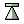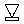*/